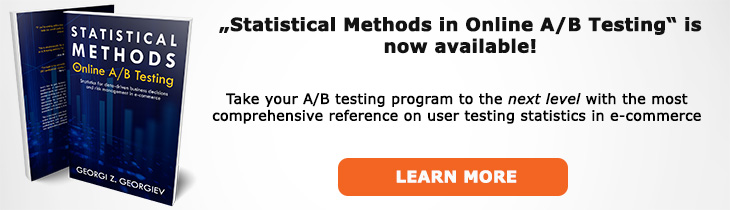# What does "Statistical Model" mean?

Definition of Statistical Model in the context of A/B testing (online controlled experiments).

## What is a Statistical Model?

In probability theory a statistical model is a mathematical model that reflects a set of statistical assumptions with regards to the process governing the generation of sample data from a larger population. The statistical model is thus an idealized form of the true data-generating mechanism (DGM). All statistical hypothesis tests and all statistical estimators are based on statistical models, including significance tests and confidence intervals.

The purpose of a statistical model is to specify the inferential premises, to allow us to assign probabilities to all events of interest (sample space), and to provide error probabilities which one can use to assess the optimality and reliability of methods for learning from data.

In a Null Hypothesis Statistical Test a hypothesis is chosen to be tested (the null hypothesis) and while it is usually communicated as a simple claim in its most complete form it is a statistical model and its statistical premises. A generic description of a statistical model is: ℳθ(x)={ƒ(x; θ), θ∈Θ}, x∈X:=ℝnX, where ƒ(x; θ), θ∈Θ is the joint distribution of the sample that encapsulates the probabilistic structure of the sample X:=(X1,...,Xn). Θ (theta) denotes the parameter space, X is the sample space and θ is the unknown parameter. The null and alternative hypotheses are then sub-spaces of the overall model (Θ01).

An example of a model used often in A/B testing is the Simple Normal Model with one parameter which has the assumption that the parameter has a normal distribution, its observations are independent observations (the result of an independent process) and identically distributed with a constant mean and variance.

The full and correct specification of the model is key since in frequentist inference learning from data occurs when the relevant error probability (type I error or type II error) relates directly to an adequate description of the underlying data-generating mechanism. If the model is not statistically adequate in the sense that its probabilistic assumptions are not valid for the data in question, it means that it could not have generated the data we observe so any conclusion we come to is then flawed if it can be interpreted at all.

Prominent statistician George Box famously remarked that "All models are false, but some are useful!" which is sometimes misunderstood by focusing on the first part and interpreting it as meaning that nothing can be learned through statistical models while completely forgetting the second part. However, the first part is a mere truism: a model is by definition an imperfect representation of a complex mechanism. The second part, however, is what is really interesting and it tells us that we can use models to guide decisions in the real world. The quote that gives the most context is in fact: "Remember that all models are wrong; the practical question is how wrong do they have to be to not be useful."  which obviously shifts the focus from the first part to the issue of mis-specification testing (testing for departures in assumptions).

References:
 Box G.E.P., Draper N.R. (1987) "Empirical Model-Building and Response Surfaces", p. 424
 Spanos A. (2011) "Foundational Issues in Statistical Modeling - Statistical Model Specification and Validation"
 Spanos A. (2013) "Frequentist Hypothesis Testing a Coherent Account"

## Related A/B Testing terms

Hypothesis

Sample Space

Statistical Design

Hypothesis Testing

Like this glossary entry? For an in-depth and comprehensive reading on A/B testing stats, check out the book "Statistical Methods in Online A/B Testing" by the author of this glossary, Georgi Georgiev.## Glossary Index by Letter

Select a letter to see all A/B testing terms starting with that letter or visit the Glossary homepage to see all.